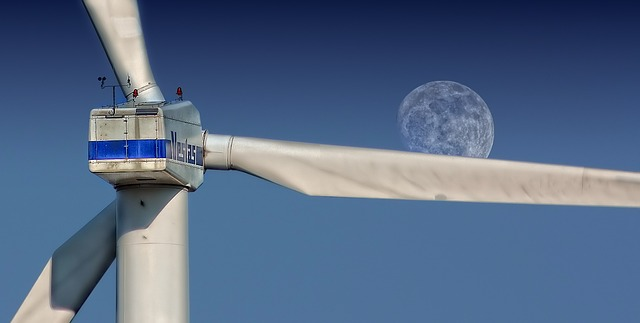µ GCSE | Power

10 quick questions - for GCSE and iGCSE

10 minutes maximum! (can you do it in 5?)

1. Which of these statements is the best definition of power?

• A. The total work done.
• B. The total work done x the time taken.
• C. The total work done x the distance travelled.
• D. The rate at which work is done.

2. Which of these statements is the best definition of work done?

• A. The energy used up by a system.
• B. The energy wasted in a system.
• C. The energy transferred in a system.
• D. The energy stored in a system.

3. Which of the following correctly gives the units used for measuring work done and power?

work done power
A
watts joules
B
watts volts
C
joules volts
D
joules watts

4. A builder carries a varying mass of bricks to the top floor of a building. Which of the following required the most power?

• A. 10kg load lifted up in 60 seconds
• B. 10kg load lifted up in 120 seconds
• C. 20kg load lifted up in 60 seconds
• D. 20kg load lifted up in 120 seconds
5. A wind turbine has a peak output of 0.15 MW.This is the same as:

6-8. Kettles are used to heat water using electrical power. The table below gives some data about 3 kettles. What are the missing values in the table?

 Type of kettle Work Done Time taken Power 6. kettle A 40 000 J 80 s ? 2 MW 500 W 2 mW 3.2 MW 7. kettle B 300 000 J 1 min ? 300 000 kW 300 000 W 5000 W 5000 kW 8. kettle C ? 80 kJ 8 000 J 50 J 20 J 40 s 2 kW

9+10. A solar panel is used to charge a phone on a camping trip.
The peak power output of the solar panel is 2 W. The phone needs 20 kJ to be fully charged.

9. How long will it take to charge the phone?

• A. 40 000 s
• B. 10 000 s
• C. 4 000 s
• D. 1000 s

10. Julie needs her phone charging in just 1 hour. She wants to buy a more powerful solar panel. What power output from the solar panel is needed?

• A. 5.6 W
• B. 3.3 W
• C. 20 kW
• D. 0.18 W